Excel Investment Calculator can calculate compound interest and provide the future value of an investment. It is a powerful tool used to determine the outcome of your investments.

### You can determine how much your money will grow using Excel Investment Calculator.

In this tutorial, you will learn:

### What is Compound Interest?

Compound interest is often referred to as “interest on interest” i.e. you earn interest on both:

• Initial investment; and
• Previous period’s interest earned

As opposed to simple interest, it is assumed that the interest earned is reinvested and in the future periods, you will be earning interest on both principal and reinvested interest (not just on principal amount). The longer you save, the more interest you will earn.

For example, you deposit \$100 for 2 years at a compound interest of 10%. In the first year, you will earn \$100*0.10 i.e. \$10 and in the second year, you will earn \$100*0.10 + \$10*0.10 i.e. 11. So, you will earn a total of \$21 in interest rather than \$20 as in the case of simple interest.

Using Excel Investment Calculator, you can easily calculate different attributes of compound interest. Let’s see how it can be done!

### Calculation using Mathematical Formula

To calculate the future value of your investment, you need to know three factors:

• PV – Present Value of Investment
• i – Annual interest rate
• n – Compounding frequency
• t – no of periods

Using these three factors, you can find out the future value of your investment with a certain compounded interest rate.

## = PV * (1 + i/n)nt

Let’s take an example to understand how this formula works in Excel.

Suppose you invest \$4000 for a period of 8 years at a monthly compound interest of 5% and you want to know the value of the investment after 8 years.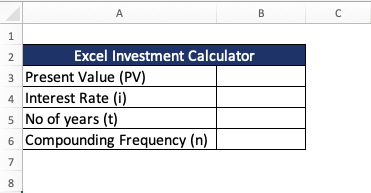STEP 1: The Present Value of investment is provided in cell B3.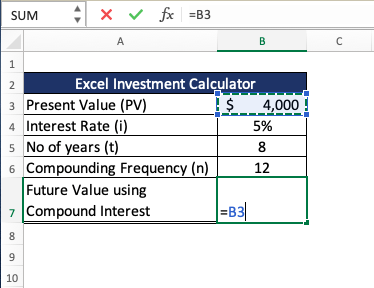STEP 2: The annual interest rate is in cell B4 and the interest is compounded monthly so the interest will be divided by the compounding frequency 12 (in cell B6).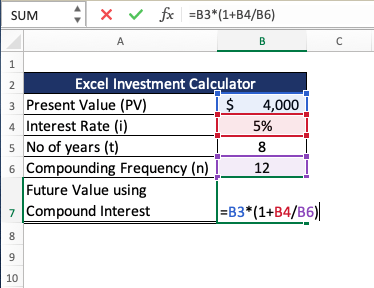STEP 3: Since compounding is done monthly, we need to multiple the no of years (cell B6) with compounding frequency (cell B5).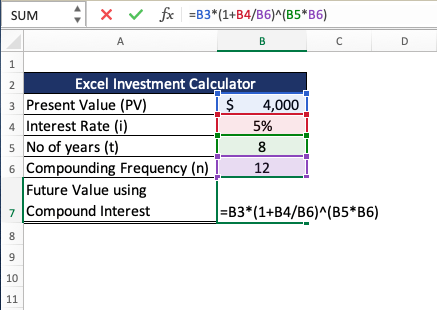Once, you have provided Excel Investment Calculator with all the necessary inputs it will calculate the FV of the investment for you which is \$5,962 in this case.

This is how your Monthly Investment Calculator Excel will look like: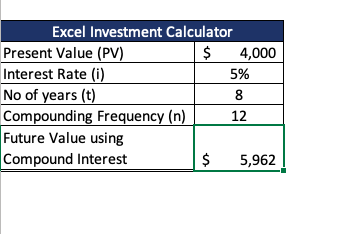If you need to calculate the future value of an interest when compounding frequency is quarterly, you can simply change the value in cell B6 to 4.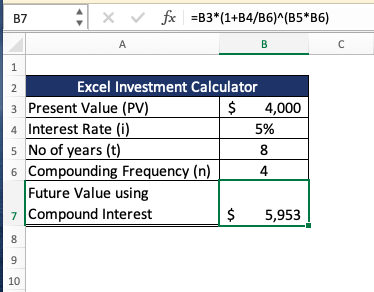### Calculation using Excel’s FV Formula

Computing the compound interest of an initial investment is easy for a fixed number of years.  But let’s add an additional challenge.

What if you are also putting in monthly contributions to your investment? Now that’s a lot more challenging to compute now!

How much would be available for you at the end of your investment?

Thankfully there is an easy way to calculate this with Excel Investment Calculator – The FV formula! FV stands for Future Value.

Formula breakdown:

=FV(rate, nper, pmt, [pv],[type])

What it means:

=FV(interest rate, number of periods, periodic payment, initial amount)

• rate – Interest rate per period
• nper – Total no of compounding periods
• pmt – Annuity amount per period. If this is omitted, make sure you provide Excel with a PV.
• [pv] – Present value of the investment. This is an optional argument.
• [type] – It is should be 0 if the annuity is received at the end of the compounding period and 1 if it received at the beginning of the compounding period. This is an optional argument and by default, its value is set to 0.

In our example below, we have the table of values that we need to get the compound interest or Future Value from using Excel Investment Calculator: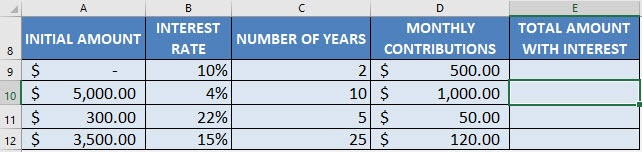There are two important concepts we need to use since we are using monthly contributions:

• Since our interest rate is the annual rate, we will have to divide it by 12 to make it monthly
• We will need to convert our number of years into a number of months by multiplying it by 12I explain how you can do this below:STEP 1: We need to enter the FV function in a blank cell:

## =FV(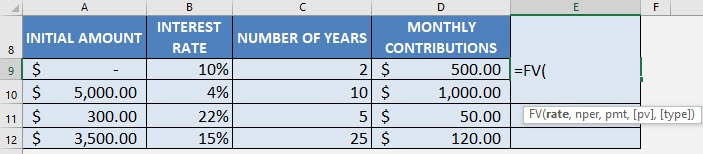STEP 2: The FV arguments:

## rate

What is the rate of interest?

Select the cell containing the interest rate and divide it by 12 to get the monthly interest rate (make sure that this is in a percentage):

## =FV(B9/12,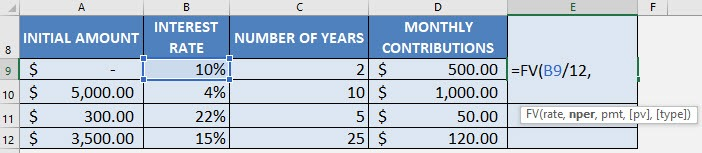## nper

How many periods?

Select the cell containing the number of years and multiply it by 12 to get the number of months:

## =FV(B9/12, C9*12,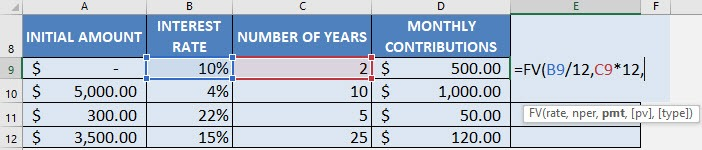## pmt

What is the periodic payment?

Select the cell that contains your monthly contribution (this is your periodic payment):

## =FV(B9/12, C9*12, D9,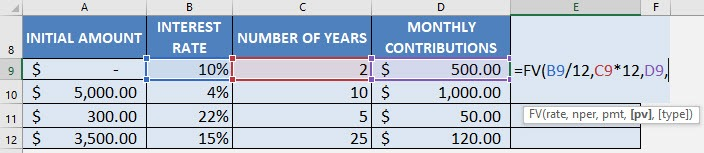## pv

What is the initial amount?

PV stands for present value, the initial amount. Multiply the entire result by -1.

## =FV(B9/12, C9*12, D9, A9) * -1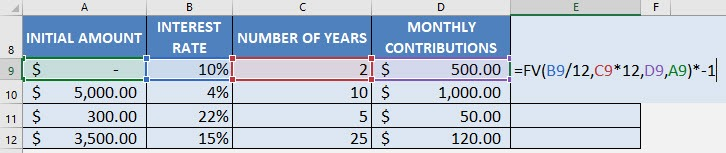Apply the same formula to the rest of the cells by dragging the lower right corner downwards.

You now have all of the compound interest results in the investment calculator Excel!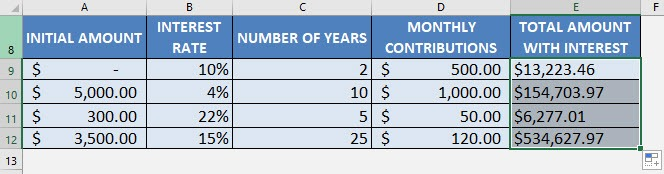### Conclusion

In this article, you have understood the concept of compounding i.e. reinvesting the interest earned on investments. Using Excel Investment Calculator, you can compute the future value of your investment by either using the mathematical formula or the FV formula.